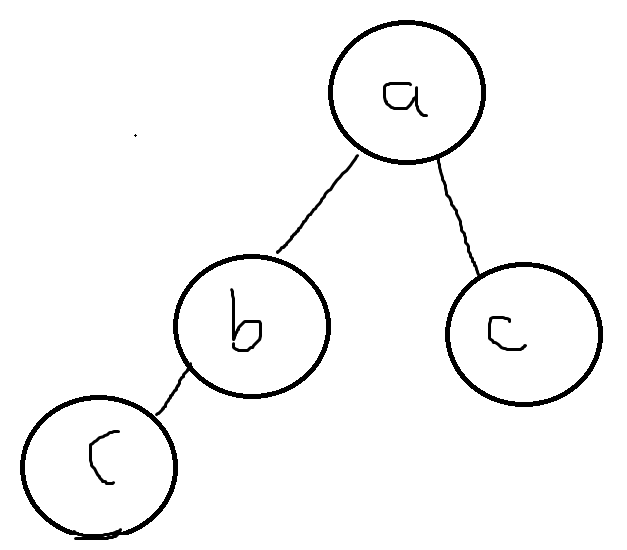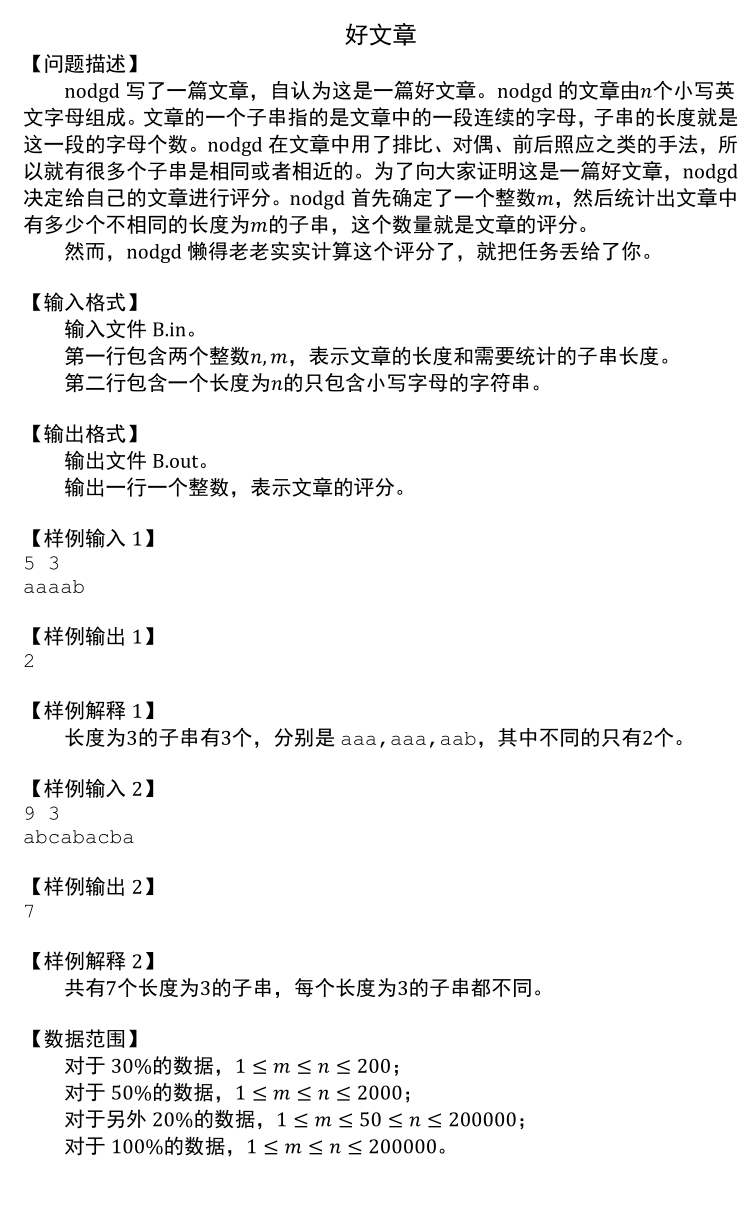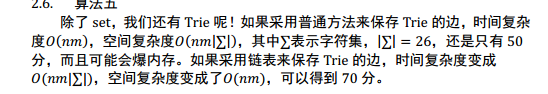# 理论知识与功能

## 定义

Trie（字典树），用于实现字符串的快速检索。其每个节点都含有若干个字符指针。## 插入

1.若P的c字符指针指向空（即没有这个节点），则新建一个节点Q，令P的c字符指针指向Q，然后令P=Q。

2.若指向一个已存在的节点，直接让P=Q。

## 检索

1.若P的c指针指向空，则S没有被插入过Trie，返回False

2.若指向存在的节点，则P=Q

## 代码实现

```int trie[Size],tot=1;
void insert(char* str){
int len=strlen(str),p=1;
for(int k=0;k<len;k++){
int ch=str[k]-'a';
if(trie[p][ch]==0){
trie[p][ch]=++tot;
}
p=trie[p][ch];
}
end[p]=true;
}
bool search(char* str){
int len=strlen(str),p=1;
for(int k=0;k<len;k++){
p=trie[p][str[k]-'a'];
if(p==0){
return false;
}
}
return end[p];
}```

# 题中的Show Time

## 好文章 2015模拟题```#include<iostream>
#include<cstring>
using namespace std;
const int Size=200100;
int trie[Size];
bool end[Size];
char S[Size];
int tot;
int ans;
int n,m;
bool Search(int pos){
//int len=strlen(str),p=1;
int len=m,p=1;
for(int k=0;k<len;k++){
p=trie[p][S[k+pos]-'a'];
if(p==0){
return false;
}
}
return end[p];
}
void Insert(int pos){//char *str
//int len=strlen(str),p=1;
if(Search(pos)){
//cout<<"重复"<<endl;
return;
}
int len=m,p=1;
for(int k=0;k<len;k++){
int ch=S[pos+k]-'a';
if(trie[p][ch]==0){
trie[p][ch]=++tot;

//cout<<"p "<<p<<endl;
}
p=trie[p][ch];
}
end[p]=true;
ans++;
}
int main(){
//abc bca cab aba bac acb cba
//string s;
//freopen("B.in","r",stdin);
//freopen("B.out","w",stdout);
cin>>n>>m>>S;
for(int i=0;i<=n-m;i++){
//cout<<"运行"<<endl;
Insert(i);
}
cout<<ans;
}```

50分解法。但由于用到了Trie，所以粘了过来。据题解所述，Trie最高能拿70分，但抱歉我没有看懂。